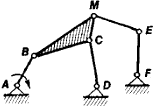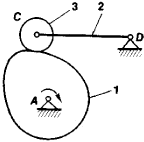# Kinematics of Mechanisms

The following article is from The Great Soviet Encyclopedia (1979). It might be outdated or ideologically biased.

## Kinematics of Mechanisms

a branch of the theory of machines and mechanisms, in which the geometric aspect of the motion of machine components or members is studied, neglecting the causes of such motion. In the kinematics of mechanisms, research is based on the assumption that any mechanism consists of movably connected rigid bodies (the members) whose motions are determined by the motion of one or more members called the driving members.

The kinematics of mechanisms solves problems of kinematic analysis and synthesis. The main problems in kinematic analysis are determination of the position of the members and of the trajectories, angular velocities, and accelerations of individual points of a mechanism. Certain constant geometric parameters of a mechanism, which define the kinematic properties of the mechanism and the laws of motion for the driving members, must be given in solving each of these problems. For example, for a plane linkwork (Figure 1), the distance between the hinge centers and the law of motion for the driving link AB must be given. For a cam mechanism (Figure 2) the shape of the cam (1) and its law of motion, the radius of the roller (3), and the distances between centers of hinges C and D and A and D must be given. The positions of the members are determined graphically or analytically.

The simpler graphic methods consist of the following steps. If for a mechanism (Figure 1) the position of the member AB and the distances between hinge centers are known, the position of all other members can be determined by intersections of circles drawn with a compass. Thus, a problem in plane mechanisms can always be reduced to a determination of points of intersection of plane curves. Graphic constructions for three-dimensional mechanisms are more complex, since they are associated with the determination of lines and points of intersection for three-dimensional shapes. However, within the accuracy limits of a graphic construction it is always possible to construct the position of all members of plane and three-dimensional mechanisms of any degree of complexity.

Analytical methods make possible definition of the positions of members with predetermined accuracy. The problem is thus reduced to the solution of a system of nonlinear equations. Computer programs have been developed for standard mechanisms.Figure 2. Cam mechanism

The trajectories of individual points of a mechanism are usually determined concurrently with the position of the members. In so doing either the graphic construction or the analytic investigation is carried out only for trajectories on whose shape the motion of the working members of the mechanism depends. The trajectories traced by points of the mechanism are highly diverse and in some cases assume the shape of complex plane or three-dimensional curves. For example, the trajectory traced by point M in Figure 1 is an algebraic curve of the sixth order. The trajectories of points located on the member ME are curves of the 14th order.

The determination of the velocities of the members and individual points of mechanisms is the most highly developed branch of kinematics of mechanisms; it uses the graphic methods of kinematic diagrams and velocity plots, as well as the analytic method. To determine the velocity of any one point, a diagram is plotted showing variations of the path of the point as a function of time. The data used in such a plot are obtained by determining the position of the members. Then, by graphic differentiation, a diagram is plotted showing variations of velocity as a function of time. This is the simplest method; however, its accuracy is low. The method using a velocity plot can be applied for plane and three-dimensional mechanisms. A velocity plot uses the relationships among velocity vectors for various points of a mechanism. The accuracy of the velocity-plot method, like that of any graphic method, is limited. Therefore, in studying mechanisms requiring higher accuracy of kinematic calculations, the use of analytic methods is preferred. Such methods can always be reduced to solution of a system of linear equations.

Accelerations of the points of a mechanism can be determined using acceleration plots or by analytic methods (solution of a system of linear equations). As a rule, kinematic diagrams are not used in determining accelerations, since the accuracy of this method depends on the accuracy of graphic differentiation of previously constructed velocity-time plots; error accumulation is possible with this type of solution. For some high-speed mechanisms a determination is carried out not only for accelerations of the first order but also for accelerations of the second order, sometimes called jerks. If a point is moving in a straight line the second-order acceleration is equal to the first derivative of the first-order acceleration or the third derivative of distance with respect to time. An acceleration of the second order is determined by plotting the jerks or by an analytic method (solution of a system of linear equations).

Problems in the kinematic synthesis of mechanisms are the inverse of the problems of kinematic analysis discussed above. In synthesis, the magnitudes to be determined are the constant parameters of a mechanism. They are determined according to given kinematic conditions—that is, according to the trajectories of some points of mechanism members and the velocities and accelerations of members and individual points. Problems of kinematic synthesis are more complex than those of kinematic analysis.

### REFERENCES

Artobolevskii, I. I. Teoriia mekhanizmov, 2nd ed. Moscow, 1967.
Dobrovol’skii, V. V. Teoriia mekhanizmov, 2nd ed. Moscow, 1953.

I. I. ARTOBOLEVSKII and N. I. LEVITSKII